Write a program to estimate the value of the percolation threshold via Monte Carlo simulation.

Install a Java programming environment. Install a Java programming environment on your computer by following these step-by-step instructions for Mac OS X, Windows, or Linux.

After following these instructions, the commands javac-algs4 and java-algs4 will classpath in algs4.jar, which contains Java classes for I/O and all of the algorithms in the textbook.

Percolation. Given a composite systems comprised of randomly distributed insulating and metallic materials: what fraction of the materials need to be metallic so that the composite system is an electrical conductor? Given a porous landscape with water on the surface (or oil below), under what conditions will the water be able to drain through to the bottom (or the oil to gush through to the surface)? Scientists have defined an abstract process known as percolation to model such situations.

The model. We model a percolation system using an n-by-n grid of sites. Each site is either open or blocked. A full site is an open site that can be connected to an open site in the top row via a chain of neighboring (left, right, up, down) open sites. We say the system percolates if there is a full site in the bottom row. In other words, a system percolates if we fill all open sites connected to the top row and that process fills some open site on the bottom row. (For the insulating/metallic materials example, the open sites correspond to metallic materials, so that a system that percolates has a metallic path from top to bottom, with full sites conducting. For the porous substance example, the open sites correspond to empty space through which water might flow, so that a system that percolates lets water fill open sites, flowing from top to bottom.)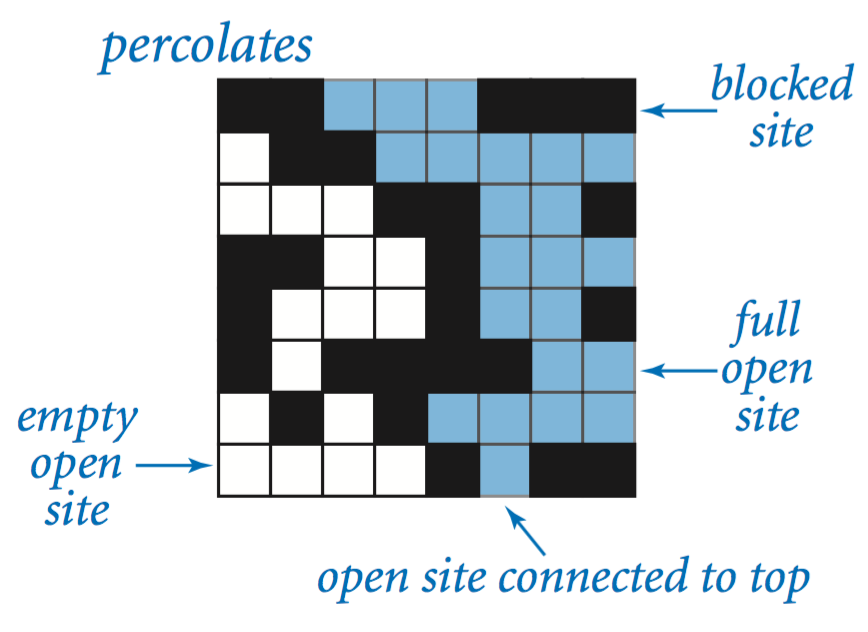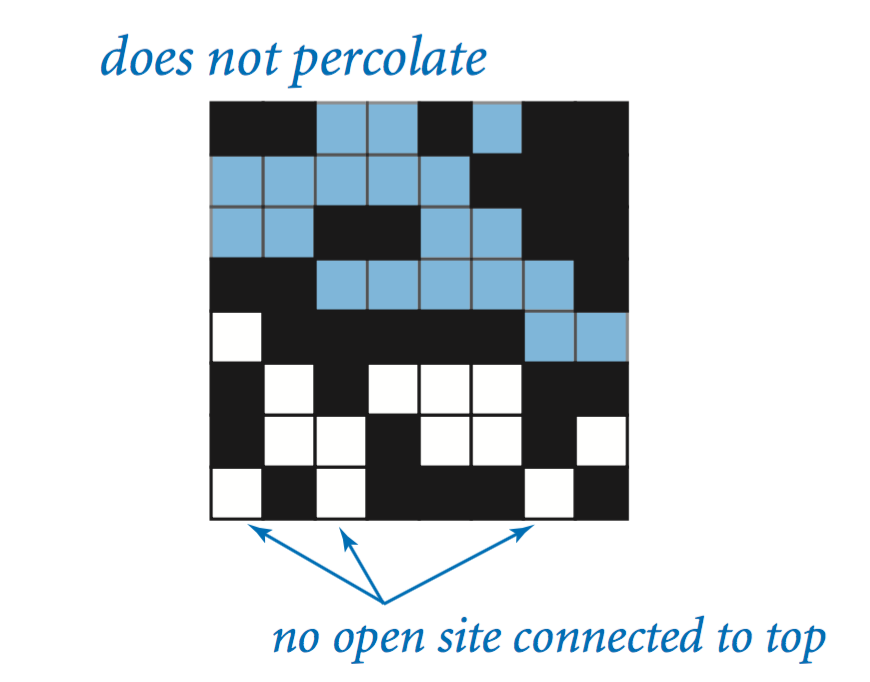The problem. In a famous scientific problem, researchers are interested in the following question: if sites are independently set to be open with probability p (and therefore blocked with probability 1 − p), what is the probability that the system percolates? When p equals 0, the system does not percolate; when p equals 1, the system percolates. The plots below show the site vacancy probability p versus the percolation probability for random 20-by-20 grids (left) and 100-by-100 grids (right).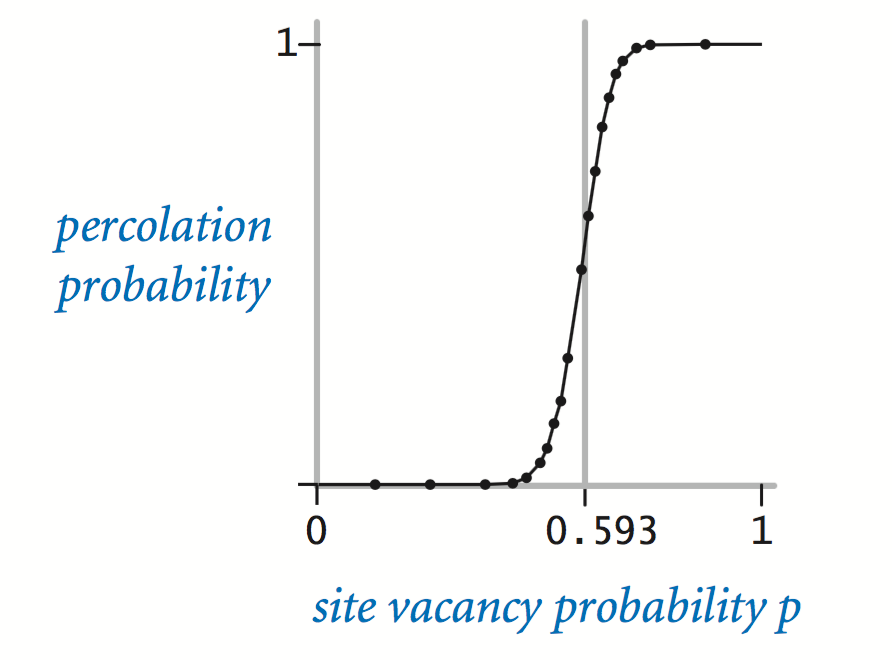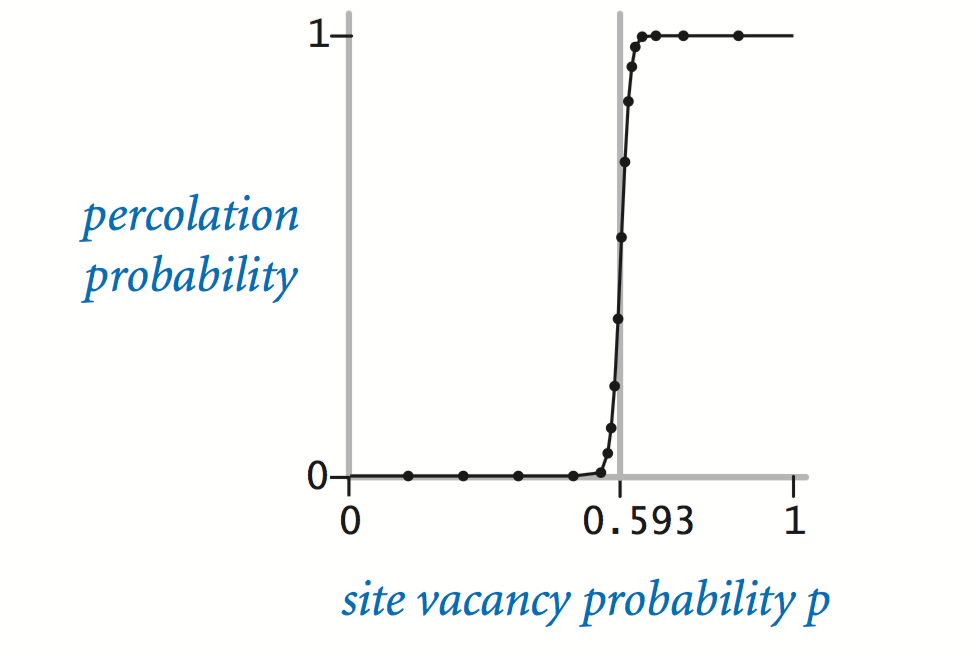When n is sufficiently large, there is a threshold value p* such that when p < p* a random n-by-n grid almost never percolates, and when p > p*, a random n-by-n grid almost always percolates. No mathematical solution for determining the percolation threshold p* has yet been derived. Your task is to write a computer program to estimate p*.

Percolation data type. To model a percolation system, create a data type Percolation with the following API:

public class Percolation {

// creates n-by-n grid, with all sites initially blocked
public Percolation(int n)

// opens the site (row, col) if it is not open already
public void open(int row, int col)

// is the site (row, col) open?
public boolean isOpen(int row, int col)

// is the site (row, col) full?
public boolean isFull(int row, int col)

// returns the number of open sites
public int numberOfOpenSites()

// does the system percolate?
public boolean percolates()

// unit testing (required)
public static void main(String[] args)

}


Corner cases.  By convention, the row and column indices are integers between 0 and n − 1, where (0, 0) is the upper-left site.

• Throw a java.lang.IllegalArgumentException if any argument to open(), isOpen(), or isFull() is outside its prescribed range.
• Throw java.lang.IllegalArgumentException in the constructor if n ≤ 0.

Unit testing.  Your main() method must call each public constructor and method directly and help verify that they work as prescribed (e.g., by printing results to standard output).

Performance requirements.  The constructor must take time proportional to n2; all methods must take constant time plus a constant number of calls to the union–find methods union(), find(), connected(), and count().

Monte Carlo simulation. To estimate the percolation threshold, consider the following computational experiment:

• Initialize all sites to be blocked.

• Repeat the following until the system percolates:

• Choose a site uniformly at random among all blocked sites.

• Open the site.

• The fraction of sites that are opened when the system percolates provides an estimate of the percolation threshold.

For example, if sites are opened in a 20-by-20 grid according to the snapshots below, then our estimate of the percolation threshold is 204/400 = 0.51 because the system percolates when the 204th site is opened.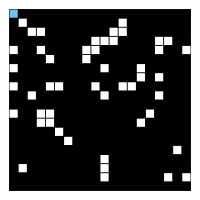50 open sites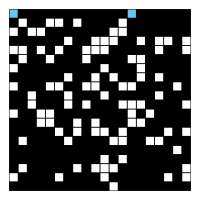100 open sites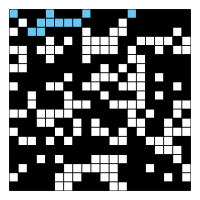150 open sites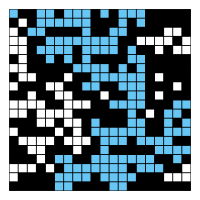204 open sites

By repeating this computation experiment T times and averaging the results, we obtain a more accurate estimate of the percolation threshold. Let xt be the fraction of open sites in computational experiment t. The sample mean $$\overline x$$ provides an estimate of the percolation threshold; the sample standard deviation s measures the sharpness of the threshold.

$\overline x = \frac{x_1 \, + \, x_2 \, + \, \cdots \, + \, x_{T}}{T}, \quad s^2 = \frac{(x_1 - \overline x )^2 \, + \, (x_2 - \overline x )^2 \,+\, \cdots \,+\, (x_{T} - \overline x )^2}{T-1}$
Assuming T is sufficiently large (say, at least 30), the following provides a 95% confidence interval for the percolation threshold:

$\left [ \overline x - \frac {1.96 s}{\sqrt{T}}, \;\; \overline x + \frac {1.96 s}{\sqrt{T}} \right]$

To perform a series of computational experiments, create a data type PercolationStats with the following API:

public class PercolationStats {

// perform independent trials on an n-by-n grid
public PercolationStats(int n, int trials)

// sample mean of percolation threshold
public double mean()

// sample standard deviation of percolation threshold
public double stddev()

// low endpoint of 95% confidence interval
public double confidenceLow()

// high endpoint of 95% confidence interval
public double confidenceHigh()

// test client (see below)
public static void main(String[] args)

}


The constructor takes two arguments n and T, and perform T independent computational experiments (discussed above) on an n-by-n grid. Using this experimental data, it calculates the mean, standard deviation, and the 95% confidence interval for the percolation threshold.

Standard libraries.  Use StdRandom to generate random numbers; use StdStats to compute the sample mean and standard deviation; use Stopwatch to measure the running time.

Corner cases.  Throw a java.lang.IllegalArgumentException in the constructor if either n ≤ 0 or T ≤ 0.

Test client.  The test client takes two command-line arguments n and T and prints the relevant statistics for T computational experiments on an n-by-n grid.

~/Desktop/percolation> java-algs4 PercolationStats 200 100
mean()           = 0.592993
stddev()         = 0.008770
confidenceLow()  = 0.591275
confidenceHigh() = 0.594712
elapsed time     = 0.373

~/Desktop/percolation> java-algs4 PercolationStats 200 100
mean()           = 0.592877
stddev()         = 0.009991
confidenceLow()  = 0.590919
confidenceHigh() = 0.594835
elapsed time     = 0.364

~/Desktop/percolation> java-algs4 PercolationStats 2 100000
mean()           = 0.666948
stddev()         = 0.117752
confidenceLow()  = 0.666218
confidenceHigh() = 0.667677
elapsed time     = 0.087


Analysis of running time. Use a “doubling” hypothesis to estimate the running time of PercolationStats as a function of both n and T.

• Implement the Percolation data type using the quick-find algorithm in QuickFindUF. Measure the running time for various values of n and T. How does doubling n affect the total running time? How does doubling T affect the total running time? Give a formula (using tilde notation) of the total running time on your computer (in seconds) as a single function of both n and T.

• Now, implement the Percolation data type using the weighted quick-union algorithm in WeightedQuickUnionUF. Answer the same questions in the previous bullet.

Project files. The file percolation.zip contains a test client and sample input files.

Deliverables. Submit only Percolation.java (using the weighted quick-union algorithm from WeightedQuickUnionUF) and PercolationStats.java. We will supply algs4.jar. Do not call any library functions except those in StdIn, StdOut, StdRandom, StdStats, Stopwatch, WeightedQuickUnionUF, and java.lang. Finally, submit a readme.txt file and answer all questions.

Grading.

file points
Percolation.java 20
PercolationStats.java12
readme.txt 8
40

Reminder: You can lose up to 4 points for poor style and up to 4 points for inadequate unit testing.

Extra credit: You can earn 2 points of extra credit for handling backwash.

This assignment was developed by Bob Sedgewick and Kevin Wayne.
Copyright © 2008.